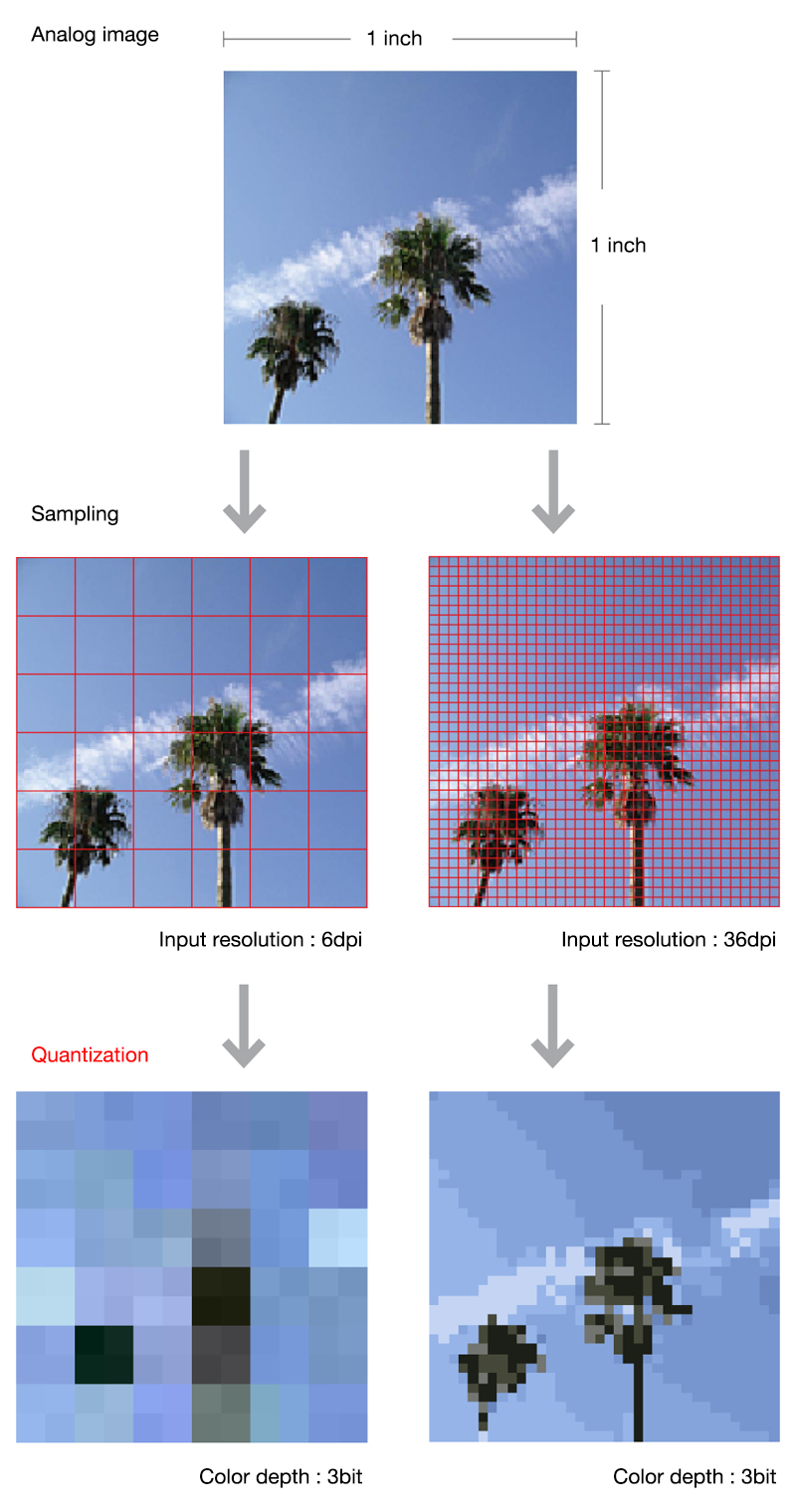# Quantization

Ryoushika

## CATEGORIES

This is the process of converting analog data such as images and audio into digital data is called analog-digital (A-D) conversion. A-D conversion is performed via two processes: sampling and quantization. Quantization is the process of converting sampled analog values into discrete digital values (values that can be represented using 0 and 1). The precision of quantization is determined by the quantization bit rate.

With images, even if the precision (the scope to be replaced with a single pixel) for measuring the analog image is decided before sampling and the color values are measured after that, the measured values must then be expressed as digital values. The quantization bit rate, which is decided at this point, dictates the number of colors that can be displayed by a single pixel (color depth). The higher the rate, that is to say, the greater the number of colors a pixel can display, the more faithfully the analog image is duplicated. (The data size increases in proportion.) If the quantization bit rate of a pixel is 1 bit, that pixel can display 2 colors. If it is 2 bits, it can display 4 colors. For example, in what is normally referred to as a “full color” digital image, the three primary colors of light (RGB) are each allocated with 8 bits (1 byte). This means that this is a 24-bit color image in which a single pixel has the quantization bit rate of 24 bits (3 bytes) and the capacity to display approximately 16.77 million colors.

With sounds, the process of deciding the number of times the sound waves are measured in a second and actually taking the measurement is carried out during sampling, but the process of determining the extent to which the measured values are expressed in quantization bit rates and converting them to digital values is carried out during quantization. Music CDs use a quantization bit rate of 16 bits. This means that the sampled values are expressed in 65,536 levels of discrete values.

In this way, it is best to visualize analog images and sounds being converted into digital data via the same processes.

READ MORE

## RELATED PAGES

• Concepts of image sampling and quantization• Concepts of audio sampling and quantization